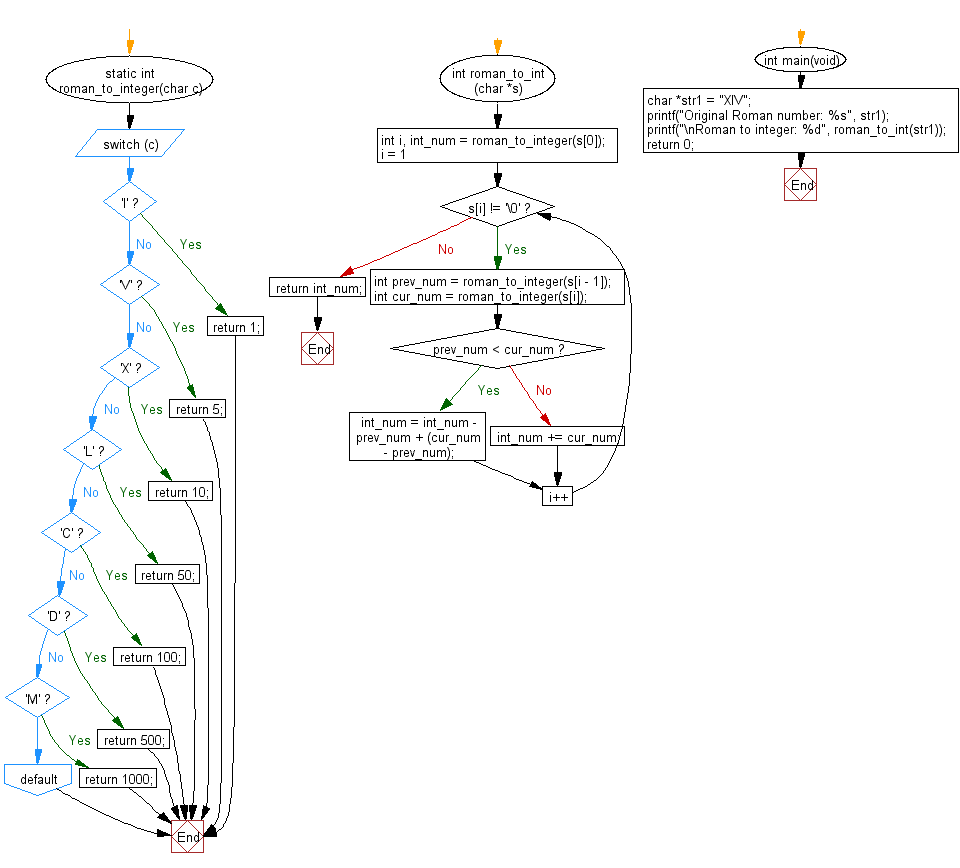﻿ C Program: Convert a given roman number to an integer - w3resource# C Exercises: Convert a given roman number to an integer

## C Programming Practice: Exercise-7 with Solution

Write a C programming to convert a given roman number to an integer.

```Roman numerals are represented by seven different symbols: I, V, X, L, C, D and M.
Symbol       Value
I             1
V             5
X             10
L             50
C             100
D             500
M             1000
```

C Code:

``````#include <stdio.h>
#include <stdlib.h>
static int roman_to_integer(char c)
{
switch(c) {
case 'I':
return 1;
case 'V':
return 5;
case 'X':
return 10;
case 'L':
return 50;
case 'C':
return 100;
case 'D':
return 500;
case 'M':
return 1000;
default:
return 0;
}
}

int roman_to_int (char *s)
{
int i, int_num = roman_to_integer(s);

for (i = 1; s[i] != '\0'; i++) {
int prev_num = roman_to_integer(s[i - 1]);
int cur_num = roman_to_integer(s[i]);
if (prev_num < cur_num) {
int_num = int_num - prev_num + (cur_num - prev_num);
} else {
int_num += cur_num;
}
}
return int_num;
}
int main(void)
{
char *str1 = "XIV";
printf("Original Roman number: %s", str1);
printf("\nRoman to integer: %d", roman_to_int(str1));
return 0;
}
``````

Sample Output:

```Original Roman number: XIV
Roman to integer: 14
```

Pictorial Presentation:Flowchart:## C Programming Code Editor:

What is the difficulty level of this exercise?

Test your Programming skills with w3resource's quiz.

﻿

## C Programming: Tips of the Day

Maximum value of int:

In C:

```#include <limits.h>
then use
int imin = INT_MIN; // minimum value
int imax = INT_MAX;```

or

```#include <float.h>

float fmin = FLT_MIN;  // minimum positive value
double dmin = DBL_MIN; // minimum positive value

float fmax = FLT_MAX;
double dmax = DBL_MAX;```

Ref : https://bit.ly/3fi8yk9